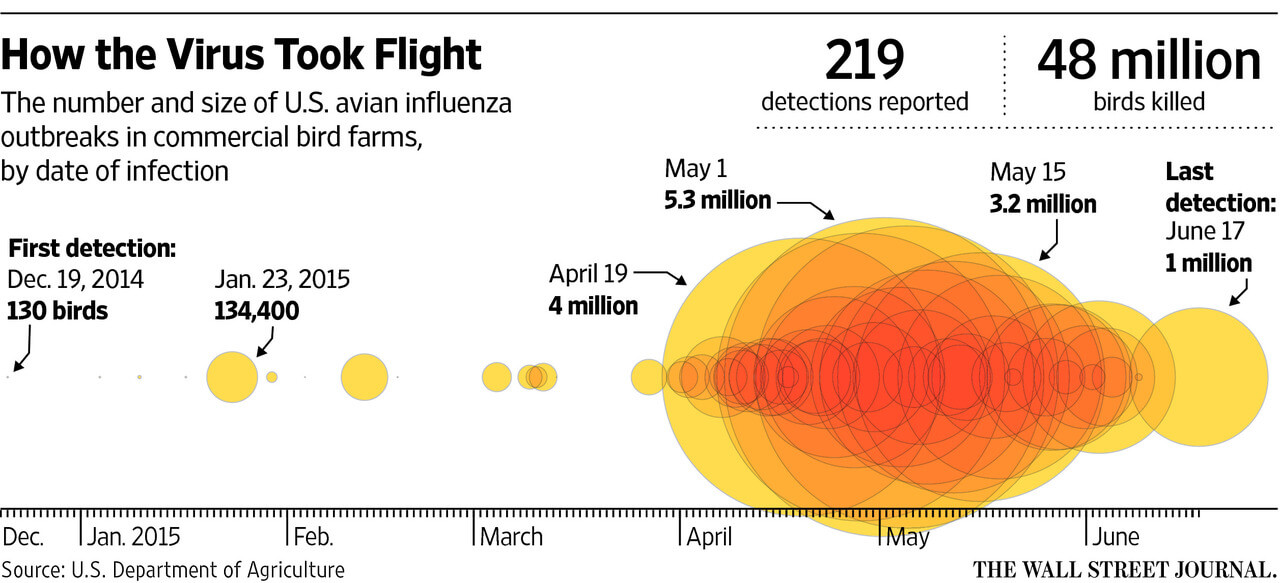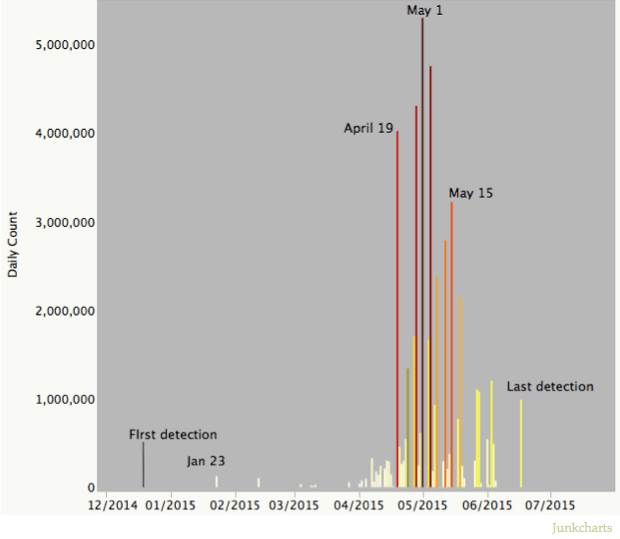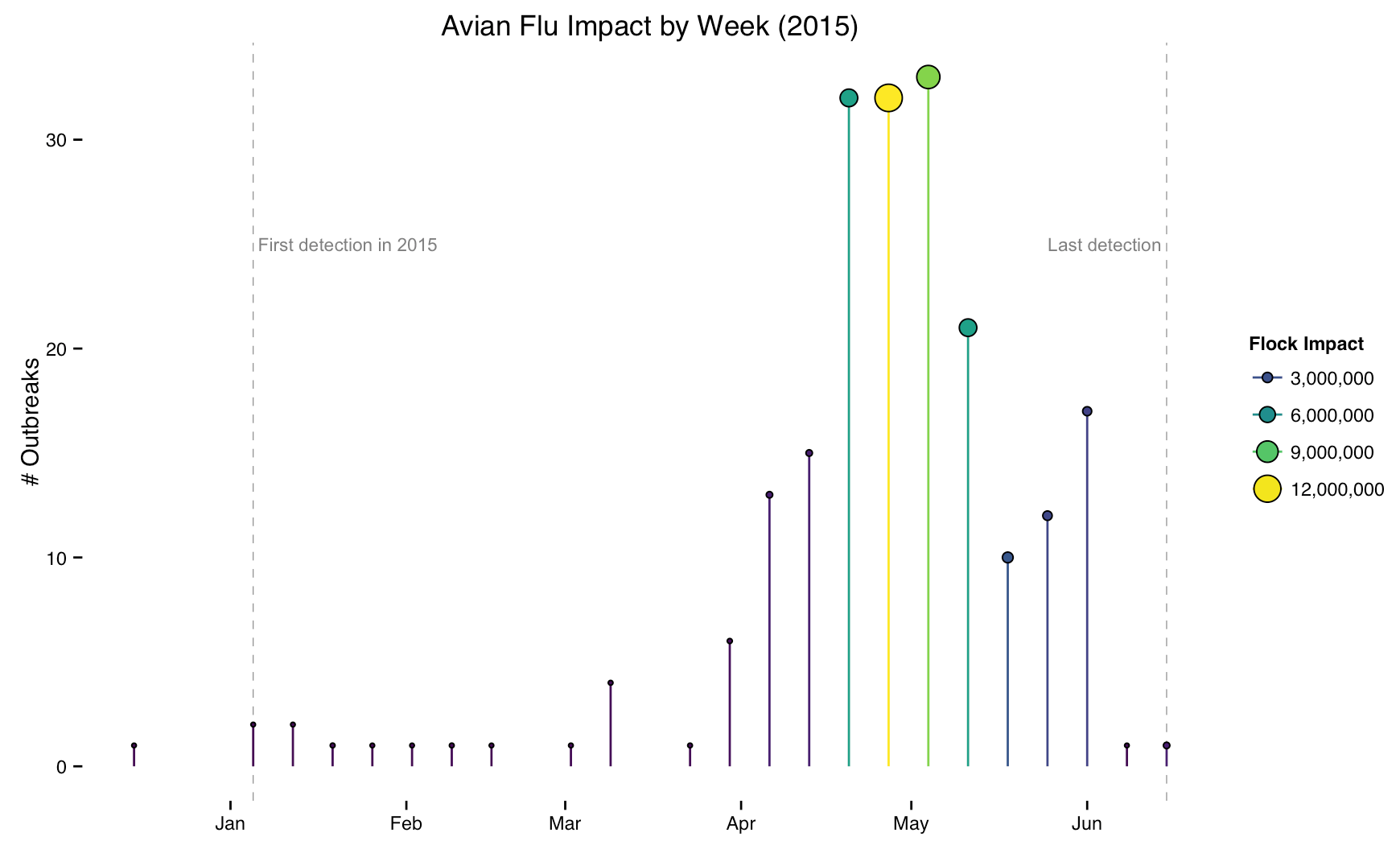Want to share your content on R-bloggers? click here if you have a blog, or here if you don't.

Junk Charts adeptly noted and fixed this excessively stylized chart from the WSJ this week:Their take on it does reduct the ZOMGOSH WE ARE DOOMED! look and feel of the WSJ chart:But, we can further reduce the drama by using a more neutral color encoding and encode both the # of outbreaks and total size of the impacted flock populations per week with a lollipop chart (and, thankfully the USDA makes this data readily available):

``` library(xml2) library(rvest) library(dplyr) library(stringr) library(ggplot2) library(scales) library(viridis) library(ggthemes)   pg <- read_html("https://www.aphis.usda.gov/wps/portal/aphis/ourfocus/animalhealth/sa_animal_disease_information/sa_avian_health/sa_detections_by_states/ct_ai_pacific_flyway/!ut/p/a1/lVNNb-IwEP0tPewx2OSbI_QDwkdBRbuQXKyJ4yTWJnYUG1D-fZ10D7RqadcHS555M_PmPRkl6IgSAWdegOZSQNW_E58stwt7PMN2NN9PHnH0_OdpE64DZ7twDSA2APzFmeL39dtV5Pf1L3i2eBjjvYMOKEEJFbrRJYqhKbkiVArNhCYVT1tou19YAZGnluSSntTwAsFrqEjJoNLldSTjioFihItctvWwxFv6zEFc4zOmGe3TiqQdURo0M62pJsBJA5TnnJK86i7Q9fwayjMU57ZPAezccnwvtdwM21aah9hcGHtuCP6Y5v_0uLHwj_S8n08XbrA2CrqhjaMHUxxMNhhH_nf1g57fdBgAtyz7MGT-ODNDnta7YLW08cpDsSEZfMli4qL9f6q2_IEsdru53xSmLejS6g1Gx5vGv6WvjB8CnxmPjp8af5ihxJNBpIqeX1HJdPgQ8VSkTmiItCxnLWtHpVQaHS-Xy-ikMhgV8oya-ncdOh23_r6E2PGqYrerD9O7u1eBlNG5/?1dmy&urile=wcm%3apath%3a%2Faphis_content_library%2Fsa_our_focus%2Fsa_animal_health%2Fsa_animal_disease_information%2Fsa_avian_health%2Fsa_detections_by_states%2Fct_ai_full_list")   dat <- html_table(html_nodes(pg, "table"))[]   dat %>% mutate(`Confirmation date` = as.Date(`Confirmation date`, "%b %d, %Y"), week = format(`Confirmation date`, "%Y-%U"), week_start = as.Date(sprintf("%s-1", week), "%Y-%U-%u") , `Flock size` = as.numeric(str_replace_all(`Flock size`, ",", ""))) %>% select(week, week_start, `Flock size`) %>% filter(!is.na(`Flock size`)) %>% group_by(week_start) %>% summarize(outbreaks=n(), flock_total=sum(`Flock size`)) -> dat   first <- dat[2,] last <- tail(dat, 1)   gg <- ggplot(dat, aes(x=week_start, y=outbreaks)) gg <- gg + geom_vline(xintercept=as.numeric(first\$week_start), linetype="dashed", size=0.2, color="#7f7f7f") gg <- gg + geom_text(data=first, aes(x=week_start, y=25), label=" First detection in 2015", hjust=0, size=3, color="#7f7f7f") gg <- gg + geom_vline(xintercept=as.numeric(last\$week_start), linetype="dashed", size=0.2, color="#7f7f7f") gg <- gg + geom_text(data=last, aes(x=week_start, y=25), label="Last detection ", hjust=1, size=3, color="#7f7f7f") gg <- gg + geom_segment(aes(x=week_start, xend=week_start, y=0, yend=outbreaks, color=flock_total), size=0.5) gg <- gg + geom_point(aes(size=flock_total, fill=flock_total), shape=21) gg <- gg + scale_size_continuous(name="Flock Impact", label=comma, guide="legend") gg <- gg + scale_color_viridis(name="Flock Impact", label=comma, guide="legend") gg <- gg + scale_fill_viridis(name="Flock Impact", label=comma, guide="legend") gg <- gg + scale_x_date(label=date_format("%b")) gg <- gg + guides(color=guide_legend(), fill=guide_legend(), size=guide_legend()) gg <- gg + labs(x=NULL, y="# Outbreaks", title="Avian Flu Impact by Week (2015)") gg <- gg + theme_tufte(base_family="Helvetica") gg <- gg + theme(legend.key=element_rect(color=rgb(0,0,0,0))) gg ```If we really want to see the discrete events, we can do that with our less-ZOMGOSH color scheme, too:

``` dat <- html_table(html_nodes(pg, "table"))[] dat %>% mutate(`Confirmation date` = as.Date(`Confirmation date`, "%b %d, %Y"), `Flock size` = as.numeric(str_replace_all(`Flock size`, ",", ""))) %>% filter(!is.na(`Flock size`)) %>% rename(date=`Confirmation date`) %>% arrange(date) -> dat   first <- dat[2,] last <- tail(dat, 1)   gg <- ggplot(dat, aes(x=date, y=`Flock size`)) gg <- gg + geom_vline(xintercept=as.numeric(first\$date), linetype="dashed", size=0.2, color="#7f7f7f") gg <- gg + geom_text(data=first, aes(x=date, y=3000000), label=" First detection in 2015", hjust=0, size=3, color="#7f7f7f") gg <- gg + geom_vline(xintercept=as.numeric(last\$date), linetype="dashed", size=0.2, color="#7f7f7f") gg <- gg + geom_text(data=last, aes(x=date, y=3000000), label="Last detection ", hjust=1, size=3, color="#7f7f7f") gg <- gg + geom_segment(aes(x=date, xend=date, y=0, yend=`Flock size`, color=`Flock size`), size=0.5, alpha=1) gg <- gg + scale_size_continuous(name="Flock Impact", label=comma, guide="legend") gg <- gg + scale_color_viridis(name="Flock Impact", label=comma, guide="legend") gg <- gg + scale_fill_viridis(name="Flock Impact", label=comma, guide="legend") gg <- gg + scale_x_date(label=date_format("%b")) gg <- gg + scale_y_continuous(label=comma) gg <- gg + guides(color=guide_legend(), fill=guide_legend(), size=guide_legend()) gg <- gg + labs(x=NULL, y="Flock size", title="Avian Flu Impact (2015)") gg <- gg + theme_tufte(base_family="Helvetica") gg <- gg + theme(legend.key=element_rect(color=rgb(0,0,0,0))) gg ```Neither of those is ever going to sell any ads, tho.